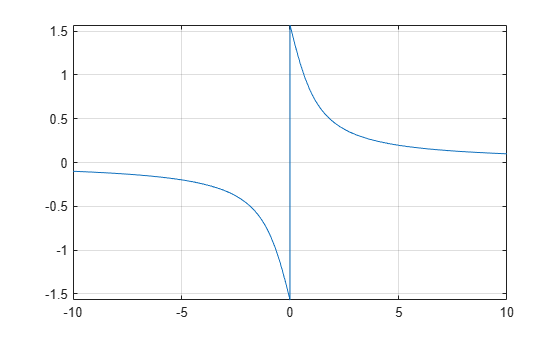# acot

Symbolic inverse cotangent function

## Syntax

``acot(X)``

## Description

example

````acot(X)` returns the inverse cotangent function (arccotangent function) of `X`. All angles are in radians. For real values of `X`, `acot(X)` returns values in the interval `[-pi/2,pi/2]`.For complex values of `X`, `acot(X)` returns complex values with the real parts in the interval `[-pi/2,pi/2]`. ```

## Examples

### Inverse Cotangent Function for Numeric and Symbolic Arguments

Depending on its arguments, `acot` returns floating-point or exact symbolic results.

Compute the inverse cotangent function for these numbers. Because these numbers are not symbolic objects, `acot` returns floating-point results.

`A = acot([-1, -1/3, -1/sqrt(3), 1/2, 1, sqrt(3)])`
```A = -0.7854 -1.2490 -1.0472 1.1071 0.7854 0.5236```

Compute the inverse cotangent function for the numbers converted to symbolic objects. For many symbolic (exact) numbers, `acot` returns unresolved symbolic calls.

`symA = acot(sym([-1, -1/3, -1/sqrt(3), 1/2, 1, sqrt(3)]))`
```symA = [ -pi/4, -acot(1/3), -pi/3, acot(1/2), pi/4, pi/6]```

Use `vpa` to approximate symbolic results with floating-point numbers:

`vpa(symA)`
```ans = [ -0.78539816339744830961566084581988,... -1.2490457723982544258299170772811,... -1.0471975511965977461542144610932,... 1.1071487177940905030170654601785,... 0.78539816339744830961566084581988,... 0.52359877559829887307710723054658]```

### Plot Inverse Cotangent Function

Plot the inverse cotangent function on the interval from -10 to 10.

```syms x fplot(acot(x),[-10 10]) grid on```### Handle Expressions Containing Inverse Cotangent Function

Many functions, such as `diff`, `int`, `taylor`, and `rewrite`, can handle expressions containing `acot`.

Find the first and second derivatives of the inverse cotangent function:

```syms x diff(acot(x), x) diff(acot(x), x, x)```
```ans = -1/(x^2 + 1) ans = (2*x)/(x^2 + 1)^2```

Find the indefinite integral of the inverse cotangent function:

`int(acot(x), x)`
```ans = log(x^2 + 1)/2 + x*acot(x)```

Find the Taylor series expansion of `acot(x)` for ```x > 0```:

```assume(x > 0) taylor(acot(x), x)```
```ans = - x^5/5 + x^3/3 - x + pi/2```

For further computations, clear the assumption on `x` by recreating it using `syms`:

`syms x`

Rewrite the inverse cotangent function in terms of the natural logarithm:

`rewrite(acot(x), 'log')`
```ans = (log(1 - 1i/x)*1i)/2 - (log(1i/x + 1)*1i)/2```

## Input Arguments

collapse all

Input, specified as a symbolic number, variable, expression, or function, or as a vector or matrix of symbolic numbers, variables, expressions, or functions.